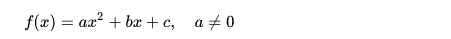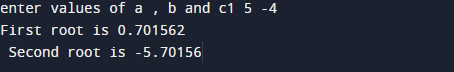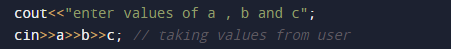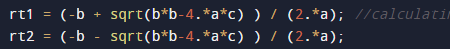C++ Program to Find All Roots of a Quadratic Equation

In this tutorial you will learn about the C++ Program to Find All Roots of a Quadratic Equation and its application with practical example.

In this tutorial, we will learn to create a C++ program that will find Second Order Quadratic Equation using C++ programming.

Prerequisites

Before starting with this tutorial we assume that you are best aware of the following C ++ programming topics:

• Operators
• Basic input/output.
• Basic C Programming
• Inbuilt library functions.

What Is Second Order Quadratic Equation?

In particular, it is a second-degree polynomial equation, since it has the greatest power is two.In algebra said a quadratic polynomial, a polynomial of degree 2.C++ Program to Find All Roots of a Quadratic Equation

In this program we will Find All Roots of a Quadratic Equation. First of all we declared and initialized the required variables.Next,we would prompt user to input the values of a,b and c then with the help of sqrt() function we will calculate the first and second root or equations.

OutputIn the above program, we have first declared and initialized a set variables required in the program.

• a,b,c = for holding coordinates
• rt1= shows first root.
• rt2= shows second root.

In this program first of we prompted user to input values of a,b and cafter getting the values of a,b and c and with the help of inbuilt function sqrt() and mathematical formula we know to calculate all Roots of a Quadratic Equation.whose value define in math.h header file we going to find the Second Order Quadratic Equation.as we know the formula of Quadratic Equation. we will use formula to get first and second root of given coordinates using c++ program.

In this tutorial we have learn about the C++ Program to Find All Roots of a Quadratic Equation and its application with practical example. I hope you will like this tutorial.